Math Worksheets Preschool Math 1st Grade Math 2nd Grade Math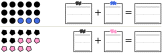Counting

Counting

Counting Chart for Bulletin Board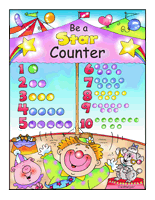"Be a Star Counter" Basics Chart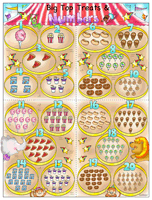"Big Top Treats & Numbers" Jumbo Chart

Counting Review Book
Counting Review Book (select age level and then print your book)

Counting Activity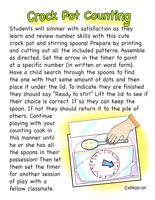Crock Pot Counting

Folding Fingers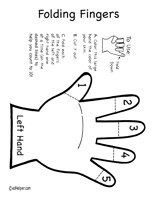Folding Fingers

Counting - Height and Length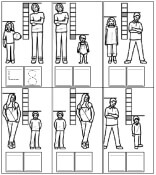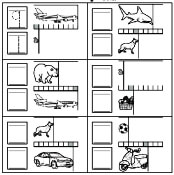Counting Shapes and Matching Totals
Total of 6 shapes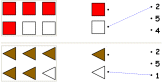Count two different colors (one answer is given)      Count two different colors

Total of 9 shapes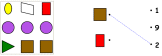Count two different colors (one answer is given)      Count two different colors      Two different shapes (one answer is given)      Two different shapes      Three different shapes (one answer is given)      Three different shapes      4-6 different shapes (one answer is given)      4-6 different shapes      3-6 different shapes with some of same shape but different colors (one answer is given)      3-6 different shapes with some of same shape but different colors

Total of 9 shapes - Similar shapes are not together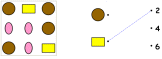Count two different colors (one answer is given)      Count two different colors      2-3 different shapes (one answer is given)      2-3 different shapes

Counting Shapes and Coloring
Total is from 4 to 8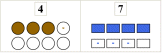Shapes to color have color spot      Only first problem has color spots      No color spots      No shapes are colored - gives total and color to use

Total is from 5 to 14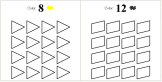Shapes to color have color spot      Only first problem has color spots      No color spots      No shapes are colored - gives total and color to use

Total is from 3 to 16 (smaller shapes and more problems per page)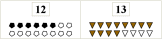Shapes to color have color spot      Only first problem has color spots      No color spots      No shapes are colored - gives total and color to use

Writing Number of Shapes and Addition of Shapes
Total is from 3 to 9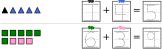Counting shapes and total both have trace numbers      One trace number given; other trace number and total are blank      All numbers blank

Total is from 5 to 9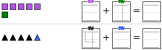Counting shapes and total both have trace numbers      One trace number given; other trace number and total are blank      All numbers blank

Total is from 10 to 15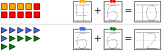Counting shapes and total both have trace numbers      One trace number given; other trace number and total are blank      All numbers blank

Total is from 10 to 20Counting shapes and total both have trace numbers      One trace number given; other trace number and total are blank      All numbers blank

Counting and Coloring Shapes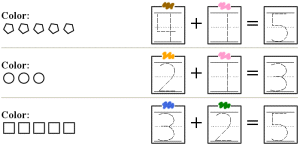Total is from 3 to 5      Numbers and total are trace numbers - color shapes      Only numbers given - color and write total Total is from 6 to 10      Numbers and total are trace numbers - color shapes      Only numbers given - color and write total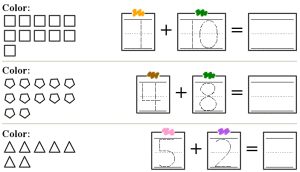Total is from 6 to 15      Numbers and total are trace numbers - color shapes      Only numbers given - color and write total Total is from 10 to 20      Numbers and total are trace numbers - color shapes      Only numbers given - color and write total

Have a suggestion or would like to leave feedback?